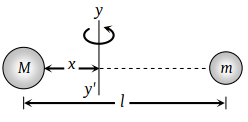# NEET Questions Solved

Two masses M and m are attached to a vertical axis by weightless threads of combined length l. They are set in rotational motion in a horizontal plane about this axis with constant angular velocity ω. If the tensions in the threads are the same during motion, the distance of M from the axis is

(1) $\frac{Ml}{M+m}$

(2) $\frac{ml}{M+m}$

(3) $\frac{M+m}{M}l$

(4) $\frac{M+m}{m}l$

(2) If the both mass are revolving about the axis yy' and tension in both the threads are equal then$M{\omega }^{2}x=m{\omega }^{2}\left(l-x\right)$

$Mx=m\left(l-x\right)$

$x=\frac{ml}{M+m}$

Difficulty Level:

• 18%
• 56%
• 22%
• 6%
Crack NEET with Online Course - Free Trial (Offer Valid Till August 23, 2019)